Next: About this document ... Up: lab_template Previous: lab_template

Subsections

# Implicit Differentiation

## Purpose

The purpose of this lab is to give you experience using Maple to compute derivatives of functions defined implicitly.

## Background

### Implicit Differentiation

The implicitdiff command can be used to find derivatives of implicitly defined functions. Suppose we wanted to use implicit differentiation to find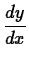for the relationThen we first define our relation and give it a label for later use.
> f:=x^2*y^2+y^3=0;

The syntax of the implicitdiff command is shown by the following example.
> implicitdiff(f,y,x);


The result of the command is the implicit derivative,. The syntax of this command is very similar to that of the diff command. The first argument is always the relation that you want to differentiate implicitly. We were careful to use an equation for this argument, but if you just give an expression for this argument, Maple assumes you want to set this expression equal to zero before differentiating. The second argument to the implicitdiff command is where you tell Maple what the dependent variable is. That is, by putting y here, we were saying that we were thinking of this relation as definingand not
. The remaining arguments to implicitdiff are for specifying the order of the derivative you want.

Second derivatives can also be computed with implicitdiff. The following command computes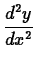.

> implicitdiff(f,y,x,x);


To compute numerical values of derivatives obtained by implicit differentiation, you have to use the subs command. For example, to find the value ofat the pointyou could use the following command.

> subs({x=1,y=-1},implicitdiff(f,y,x));


Suppose you wanted to find the equation of the tangent line to the graph of
at the point. You may want to label the output toat the pointas
for slope and then you can use the point-slope form of a line to get the equation of the tangent line. The Maple commands below show how this can be done.

> m:=subs({x=1,y=-1},implicitdiff(f,y,x));
> tanline := y-(-1)=m*(x-1);


Sometimes you want the value of a derivative, but first have to find the coordinates of the point. More than likely, you will have to use the fsolve command for this. However, to get the fsolve command to give you the solution you want, you often have to specify a range for the variable. Being able to plot the graph of a relation can be a big help in this task, so we now describe the implicitplot command. This Maple command for plotting implicitly defined functions is in the plots package which must be loaded before using the command.

> with(plots):

Here is an example of using this command to plot the hyperbola
. Note that you have to specify both an
range and a
range. This is because the implicitplot command works by setting up a grid inside the ranges you specify and then using the grid points as starting values in solving the relation numerically.
> implicitplot(x^2-y^2=1,x=-3..3,y=-3..3);

To get a good graph with this command, you usually have to experiment with the ranges. For example the following command
> implicitplot(f,x=-1..1,y=1..2);

produces an empty plot. The reason is simply that there are no solutions to
with
. This is easy to see if you rewrite the equation asand recognize that both sides of the equation must be nonnegative. Usually a good strategy to follow is to start with fairly large ranges, for example
to
for both variables, and then refine them based on what you see.

This command can also have problems if the relation in question has solution branches that cross or are too close together. For example, try the following command.

> implicitplot(f,x=-1..1,y=-1..0);

Forless than about
, you should see the two smooth curves. However, for values ofcloser to zero the two curves become jagged. To understand this, we need to take a closer look at the relation we tried to plot. The key is to notice that we can factor out
and write our relation as follows.This makes it clear that the graph of the relation really has two pieces:
and. These two curves intersect at the origin, which explains why implicitplot has problems there.

As our last example, consider the relation
. Try the following commands to see what a part of the graph of this relation looks like.

> g := x^2*sin(y)=1;
> implicitplot(g,x=-4..4,y=-10..10);

Suppose you were asked to find the slope of the graph of this relation at
, but you were only given that the value ofwas about 9. Using the plot, it is relatively easy to find this derivative by first using fsolve to find thevalue and then substituting into the formula for the derivative. Note the use of a label so we can use the value ofin the next command.
> y_sol := fsolve(subs(x=2,g),y=8..10);
> evalf(subs({x=2,y=y_sol},implicitdiff(g,y,x)));


## Exercises

1. Given the relation
,
1. Plot the graph of the relation over the interval
and
.
2. Find allvalues on the graph where.
3. Find the slope at the point whereis positive and label the slope2. For the relation given in exercise 1, find the equation of the line tangent to the graph at the point whenandis positive. Plot the relation and the tangent line on the same graph.

3. For the relation
, find the second derivativeat all points where
.Next: About this document ... Up: lab_template Previous: lab_template
William W. Farr
2002-11-19